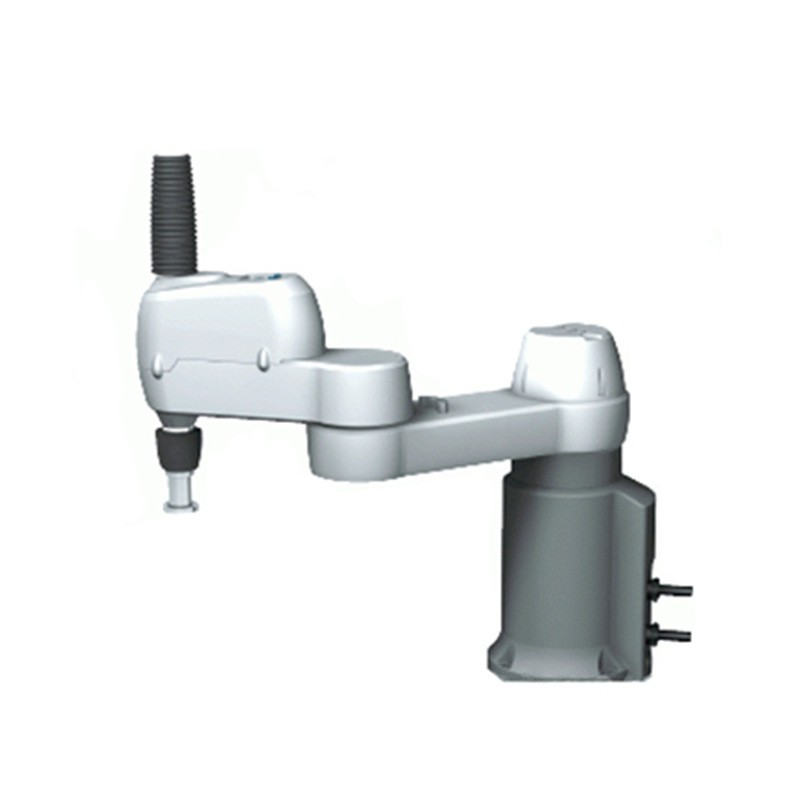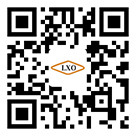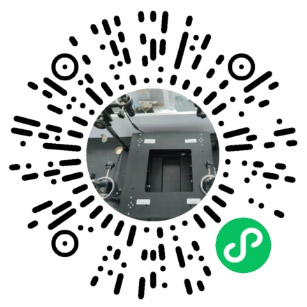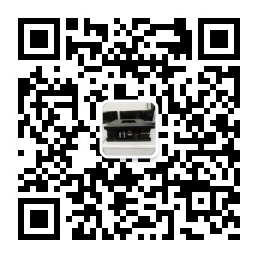4新闻中心

# 机器视觉旋转中心标定原理，ccd软件控制设定

• 机器人与视觉标定

• 1.相机固定不动, 上往下看引导机器人移动
2.相机固定不动, 下往上看
3.相机固定在机器人上，离旋转中心较近
4.相机固定在机器人上，离旋转中心很远
5.特殊固定方式 – 分离轴
目录
1.相机固定不动, 上往下看引导机器人移动

• 1.相机固定不动, 上往下看引导机器人移动
1.相机非线性校正 使用标定板做非线性校正
2.相机与机器人做9点标定 可以使用机器人扎9个点，或者机器人抓住工件摆放9个位置，得到9个 机械坐标，相机也得到9个像素坐标，然后标定

• 3.计算机器人的旋转中心 机器人抓取工件分别旋转三个角度摆放到相机视野内，相机可以得到三 个坐标值，通过三个坐标值拟合圆获得圆心坐标即为旋转中心
4.相机通过公式计算得出最终的输出结果 (rx0, ry0)为旋转中心，( x, y)为被旋转的点，(x0,y0)旋转后的点 x0= cos (a) * (x-rx0)  – sin (a) * (y-ry0) +rx0 y0= cos (a) * (y-ry0)  +  sin (a) * (x-rx0) +ry0
2.相机固定不动, 下往上看
2.相机固定不动, 下往上看
1.相机非线性校正 使用机器人吸起标定板做非线性校正
2.相机与机器人做9点标定 可以使用实物标定，机器人抓住工件摆放9个位置，得到9个 机械坐标，相机也得到9个像素坐标，然后标定。
3.计算机器人的旋转中心
4.相机通过公式计算得出最终的输出结果

• 3.相机固定在机器人上，离旋转中心较近
3.相机固定在机器人上，离旋转中心较近
1.相机非线性校正
2.相机与机器人做9点标定 可以使用实物标定，机器人抓住工件摆放9个位置，得到9个 机械坐标，相机也得到9个像素坐标(相机每次需要回到固定位置 拍照)，然后标定。
3.计算机器人的旋转中心 机器人抓取工件分别旋转三个角度摆放到相机视野内，相机 可以得到三个坐标值，通过三个坐标值拟合圆获得圆心坐标即为 旋转中心
3.相机固定在机器人上，离旋转中心较近
4.旋转中心赋值到“图案原点”
4.相机固定在机器人上，离旋转中心很远
1.相机非线性校正
2.相机与机器人做9点标定
3.计算机器人的旋转中心

• 4.相机通过公式计算得出最终的输出结果
注：由于旋转中心距离视野很远，通常拟合出来的旋转中心存在比较 大的误差，给定位精度造成影响
5.分离轴
分离轴的设计方式有很多， XY + θ, X+Y θ,   X θ+Y…., 具体状况具体分析， 目的是要找到旋转中心， 做好9点 标定。
1.相机非线性校正
2.相机与机器人做9点标定
3.计算机器人的旋转中心
4.相机通过公式计算得出最终的输出结果
不用旋转中心方法

• 旋转中心标定说明
x
y
z
Base Frame
红色框为CCD FOV，黄色为工件 五星为Mark点 训练的标准位置
工件发生平移
工件发生平移和旋转
旋转中心方法用于所有机器人与视觉配合场景
方法：计算工件实际发生的偏移量和旋转量，结合机器人的旋转中心进行二次补偿后，把补偿量 发送给机器人，然后机器人把补偿量补偿后进行抓取或放置即可；
点坐标旋转方法
计算某个点绕另外一点旋转一定角度后的坐 标，如图：A（x，y）绕B（rx0，ry0)旋转 a度后的位置为C（x0，y0），则有如下关系 式：
x0= cos (a) * (x-rx0)  – sin (a) * (y-ry0) +rx0 y0= cos (a) * (y-ry0)  +  sin (a) * (x-rx0) +ry0
下面计算所有的旋转和偏移量均是基于上面的公式
• 工件发生平移
R
Cx,Cy
MDy
MDx
• {Cx,Cy,}旋转中心坐标；{Mx,My,}工件Mark坐标 • {MDx,MDy},Mark偏移量； • {RDx,Rdy},发送给机器人的补偿量
• 工件发生平移时 • 在拍照位置进行拍照后，与开始训练标准位置 时，Mark点成像会产生偏移； • Mark偏移量MDx = RDx ; MDy = RDy;如左上图 即相机计算出的Mark点偏移量，可以直接补偿 给机器人，然后进行抓取即可
旋转中心

• 工件发生平移
R Cx,Cy R Cx,Cy
R
NCx,NCy
MDy
MDx
CDy
CDx
NCx,NCy
• 工件发生平移时 • 在拍照位置进行拍照后，与开始训练标准位置 时，Mark点成像会产生偏移； • Mark偏移量MDx = CDx ; MDy = CDy;如左上图 即相机计算出的Mark点偏移量，可以直接补偿 给机器人，然后进行抓取即可 • 作为测试，可以机器人在拍照位置进行补偿后， Z轴不变然后再拍照，会获得和拍照位置一样 的图像；
旋转中心
补偿后，旋转中心位置
旋转中心标定说明
• 工件发生平移和旋转
R
Cx,Cy
R
Cx,Cy
MDy MDx
RDy
RDx
CDr

• 工件发生平移和旋转时 • 在拍照位置进行拍照后，与开始训练 标准位置时，Mark点成像会产生偏移； • Mark偏移量MDx RDx ; MDy RDy;如 左上图即相机计算出的Mark点偏移量， 不能直接补偿给机器人，需要计算出 RDx和RDy,和RDr = MDr 然后补偿给机 器人，机器人补偿后才能进行抓取
旋转中心
• 工件发生平移和旋转
R
Cx,Cy
R
Cx,Cy
R
NCx,NCy
MDy MDx
CDy
CDx
NCx,NCy
MDr
CDr

• 工件发生平移和旋转时 • 在拍照位置进行拍照后，与开始训练标准位置时， Mark点成像会产生偏移； • Mark偏移量MDx CDx ; Mdy CDy;如左上图即相机计 算出的Mark点偏移量，不能直接补偿给机器人，需要 计算出CDx和Cdy,和CDr = MDr 然后补偿给机器人，机器 人补偿后才能进行抓取 • 作为测试，可以机器人在拍照位置进行补偿后，Z轴不 变然后再拍照，会获得和拍照位置一样的图像；
TCx,TCy
旋转中心
补偿后旋转中心
R
旋转中心标定说明—计算补偿 • 计算过程,旋转角度a
X0,Y0
X1,Y1
Cx0,Cy0
Cx1,Cy1
X0,Y0
X1,Y1
Cx0,Cy0
TCx1,TCy1
Cx1,Cy1

• 这种补偿方式是机器人先进行平移和旋转，使得机器人的姿态根据产品的当 前位置做调整，保证取料时相对关系一致，这样机器人拿到物料的姿态都是 一致的。后续做什么作业都可以。 图中黑色表示模板位置，红色表示实时物料位置，Cdx和cdy表示为了将机器 人姿态调整到与训练时一致，需要的移动量；
旋转中心标定—计算补偿 • 计算CDx = Cx1 – Cx0 和 CDy = Cy1 – Cy0
CDx= cos (a) * (Cx0-X0) - sin (a) * (Cy0-Y0) + X1 – Cx0
CDy= cos (a) * (Cy0-Y0) +  sin (a) * (Cx0-X0) + Y1 – Cy0 从公式中可以看出，补给机器人的补偿量可以由：
旋转中心坐标（Cx0,Cy0）
标准的工件(Mark)坐标点（X0,Y0）
工件移动后新的（Mark）坐标点(X1,Y1)
工件(Mark)旋转角度a
所以利用旋转中心方法计算给机器人的补偿量时，首先要计算机器人的旋转 中心坐标，但是在很多场景下计算旋转中心坐标时，要么出了视野，要么旋 转的角度范围太小等，拟合的旋转中心都存在误差，导致补偿精度不够
一定要计算旋转中心坐标吗？其实不需要
旋转中心标定—计算补偿 • CDx Cdy计算过程,旋转角度a
X0,Y0
X1,Y1
Cx0,Cy0
TCx1,TCy1 Cx1,Cy1
CDx= cos (a) * (Cx0-X0) - sin (a) * (Cy0-Y0) + X1 – Cx0 = (cos (a) -1) * (Cx0-X0)  - sin (a) * (Cy0-Y0)  + MDx = (cos (a) -1) * StDx- sin (a) * StDy+ MDx
CDy= cos (a) * (Cy0-Y0) +  sin (a) * (Cx0-X0) + Y1 – Cy0 = (cos (a) -1) * (Cy0-Y0)  + sin (a) * (Cx0-X0)  + Mdy = (cos (a) -1) * StDy + sin (a) * StDx + Mdy
从公式中可以看出StDx = Cx0-X0,StDy = Cy0-Y0是个常量，MDx和Mdy是每次拍照是工件 （Mark）的坐标与标准位置工件（Mark）的差值；
StDx与StDy怎么计算呢？蓝色的为一个二元一次方程，我们需要旋转一个角度即可计算出；
如果计算出StDx和StDy则在运行时，直接带入上述公式，可以很快计算出CDx,Cdy这样给出 Robot的偏移量了，而不需要计算旋转中心的确切坐标了；
下面介绍如何求解StDx和StDy
旋转中心标定—计算补偿 • StDx,StDy计算过程,首先Robot在拍照点旋转一定角度a（一定是Robot给出）
X0,Y0
X1,Y1

• Cx0,Cy0
TCx1,TCy1 Cx1,Cy1
CDx= cos (a) * (Cx0-X0) - sin (a) * (Cy0-Y0) + X1 – Cx0 = (cos (a) -1) * (Cx0-X0)  - sin (a) * (Cy0-Y0)  + MDx = (cos (a) -1) * StDx- sin (a) * StDy+ MDx
CDy= cos (a) * (Cy0-Y0) +  sin (a) * (Cx0-X0) + Y1 – Cy0 = (cos (a) -1) * (Cy0-Y0)  + sin (a) * (Cx0-X0)  + Mdy = (cos (a) -1) * StDy + sin (a) * StDx + Mdy
下面介绍如何求解StDx和StDy，旋转一定角度a后MDx和Mdy则为确定值，cos(a)和sin(a)为确 定值
0= (cos (a) -1) * StDx- sin (a) * StDy+ MDx
0= (cos (a) -1) * StDy + sin (a) * StDx + Mdy
StDx = -0.5*(Mdx*(cos(a)-1)+Mdy*sin(a)) / (1-cos(a))
StDy =  0.5*(Mdx*sin(a)-Mdy*(cos(a)-1))/(1-cos(a))
a是旋转标准的角度
Mdx=X1-X0，即旋转后Mark坐标与训练模板 的mark坐标
特别注意：
这里计算到的Cdx和Cdy一定要补偿到机器人的取料位置里，不能补偿到 放料位置。旋转中心方法计算到的偏差可以直接补偿到放料位置。

• 原因：
X
Y
dx
dy 旋转中心法的计算：
(X,Y)是定位到的产品位置 (GX,GY)是标准模板位置 (X’,Y’)是补偿了角度后的新位置 X’ = cos θ * (X-Xo) – sin θ *(Y-Yo) + Xo; Y’ = cos θ* (Y-Yo)  + sin θ* (X-Xo) + Yo;
Offset X=X’-GX Offset Y=Y’-GY Offset Theta= θ
(X’, Y’)
(Xo ,Yo)
(X, Y)
(GX, GY)
STD方法的计算：
CDx= cos (a) * (Cx0-X0) - sin (a) * (Cy0-Y0) + X1 – Cx0
CDy= cos (a) * (Cy0-Y0) +  sin (a) * (Cx0-X0) + Y1 – Cy0
X’= cos (a) * (X0-Cx0) - sin (a) * (Y0-Cy0) + Cx0
Y’= cos (a) * (Y0-Cy0) - sin (a) * (X0-Cx0) + Cy0
CDx=X1-X’

• Cdy=Y1-Y’
这里是机器人在取料之前，先把自己的角度补正到与 物料当前角度一致，到(X’,Y’)位置处，然后移动 CDx,Cdy，与物料位置也重合，然后去取料。
这样就保证了每次取料后，物料相对于机器人的位置 一致，因此直接往目标位置放就可以。
这种方式由于是取物料的时候调整位置，因此适用于 相机固定在机械手上、相机固定安装从上往下看的方 式。如果相机固定安装从下往上看，由于机器人这时 候已经取完料了，就不适合使用了。
X
Y
CDx
CDy
(X’, Y’)
(Cxo ,Cyo)
(X1, Y1)
(X0, Y0)
a

• 程序说明
特征点的像素坐标 机器人坐标标定完成矩阵
利用上面公式计算STDX,STDy
训练标准位置的像素坐标，需要手动输入
实际运行位置的像素坐标和机器人坐标
计算补偿量
求旋转中心方法
旋转中心
FOV
• 当相机FOV与旋转中心很近，可以用旋转3 点以上拟合一个圆求圆心

• 旋转中心
FOV
• 当相机FOV与旋转中心很远，使用旋转3或 多个点求圆心，准确性会很差。
怎么办？
旋转中心的计算偏差
旋转中心
• (X0,Y0)为旋转中心, (X1,Y1)及(X2,Y2)为 工件在视野中旋转角度a的前后坐标， (Xt,Yt)为两点连线的延长。 • d = √(X2-X1)^2 + (Y2-Y1)^2 • 角度a • R = d/2/sin(a/2) R/d = (Xt-X1)/(X2-X1) R/d = (Yt-Y1)/(Y2-Y1) Xt = (1-R/d)*X1 + (R/d)*X2 Yt = (1-R/d)*Y1 + (R/d)*Y2
a
X1,Y1
X2,Y2
Xt,Yt
X0,Y0
X0= cos (90-a/2) * (Xt-X1)  – sin (90-a/2) * (Yt-Y1) +X1 Y0= cos (90-a/2) * (Yt-Y1)  +  sin (90-a/2) * (Xt-X1) +Y1 角度：X+  Y+ 为正，反之为负
这段程序用于计算旋转中心
训练标准位置的像素坐标，需要手动输入
实际运行位置的像素坐标和机器人坐标

• 最终的补偿• 关于联勖

公司简介 公司实力 荣誉证书 视频中心
• 产品中心

视觉对位系统 视觉检测系统 对位平台 对位组件
• 解决方案

3C行业 锂电行业 家电行业 汽车行业
• 新闻中心

公司动态 行业资讯 常见问题
• 在线联系

联系我们 在线留言
•扫一扫，手机浏览扫一扫，进入小程序扫一扫，关注公众号